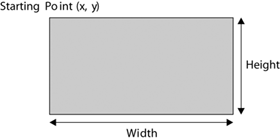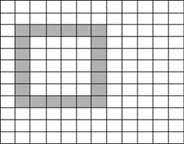# Rectangles and Regions

In previous chapters we discussed rectangles and how to draw and fill them using the draw and fill methods of the Graphics class. In this chapter we will discuss additional functionality of rectangles and regions.

We will cover the following key topics:

• .NET Framework objects that work with rectangles and regions and their members
• Graphics class members that work with rectangles and regions
• Writing applications using objects
• The Rectangle structure and its members
• The Region class and its members
• Invalidating and clipping regions
• Examples of real-world applications using regions and rectangles

A rectangle has three properties: starting point, height, and width. Figure 6.1 shows these properties where the starting point is the top left.

Figure 6.1. A rectangleSuppose you wanted to draw a rectangle from point (1, 2) with height 7 and width 6. The final rectangle would look like Figure 6.2.

Figure 6.2. A rectangle with starting point (1, 2), height 7, and width 6The filled rectangle occupies the entire area within the range of its height and width.GDI+ Programming with C#
ISBN: 073561265X
EAN: N/A
Year: 2003
Pages: 145